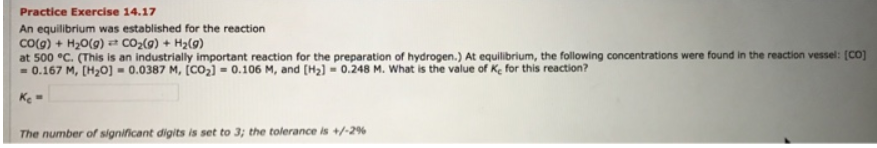# Problem: An equilibrium was established for the reaction CO(g) + H2O(g) ⇌ CO2(g) + H2(g) at 500 °C. (This is an industrially important reaction for the preparation of hydrogen.) At equilibrium, the following concentrations were found in the reaction vessel: [CO] = 0.167 M, [H2O] . 0.0387 M, [CO2] = 0.106 M, and [H2] = 0.248 M. What is the value of Kc for this reaction?

###### FREE Expert Solution###### Problem Details

An equilibrium was established for the reaction

CO(g) + H2O(g) ⇌ CO2(g) + H2(g)

at 500 °C. (This is an industrially important reaction for the preparation of hydrogen.) At equilibrium, the following concentrations were found in the reaction vessel: [CO] = 0.167 M, [H2O] . 0.0387 M, [CO2] = 0.106 M, and [H2] = 0.248 M. What is the value of Kc for this reaction?